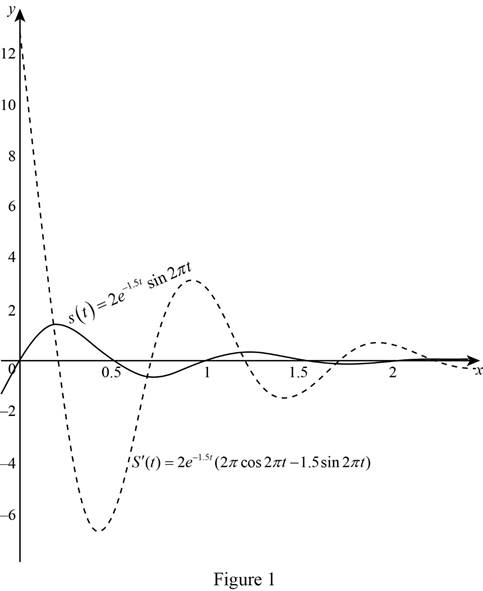# The velocity after t seconds .### Single Variable Calculus: Concepts...

4th Edition
James Stewart
Publisher: Cengage Learning
ISBN: 9781337687805### Single Variable Calculus: Concepts...

4th Edition
James Stewart
Publisher: Cengage Learning
ISBN: 9781337687805

#### Solutions

Chapter 3.4, Problem 73E
To determine

## To find: The velocity after t seconds.

Expert Solution

The velocity after t seconds is s(t)=2e1.5t(2πcos2πt1.5sin2πt)_.

### Explanation of Solution

Given:

The equation of motion of a point on such a spring is s(t)=2e1.5tsin2πt.

Derivative rule:

(1) Constant Multiple Rule: ddx[cf(x)]=cddx[f(x)]

(2) Product Rule: ddx(fg)=fddx(g)+gddx(f)

Recall:

If x(t) is a displacement of a particle and the time t is in seconds, then the velocity of the particle is v(t)=dsdt.

Calculation:

Obtain the velocity at time t.

v(t)=ddx(s(t))=ddx(2e1.5tsin2πt)

Apply the product rule (2) and the constant multiple rule (1),

v(t)=2e1.5tddx(sin2πt)+sin2πtddx(2e1.5t)=2e1.5t(cos2πt)+2sin2πt(1.5e1.5t)=2(2πe1.5t(cos2πt)1.5sin2πte1.5t)=2e1.5t(2π(cos2πt)1.5sin2πt)

Therefore, the velocity after t seconds is s(t)=2e1.5t(2πcos2πt1.5sin2πt)_.

To sketch: The position and velocity functions for 0t2

Graph:

Using the online graphing calculator and the draw graph below shown in Figure 1.Observation:

From Figure 1, it is noticed that

(i) If S(t) is positive, then S(t) is an increasing function.

(ii) If S(t) is negative, then S(t) is a decreasing function.

(iii) If S(t) is local extreme (that is, local minimum or local maximum), then S(t) crosses the x-axis (S(t)=0).

### Have a homework question?

Subscribe to bartleby learn! Ask subject matter experts 30 homework questions each month. Plus, you’ll have access to millions of step-by-step textbook answers!